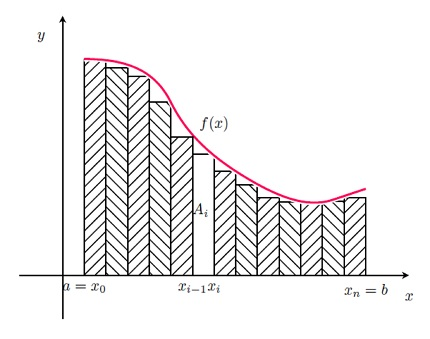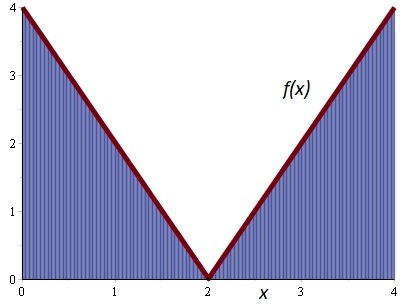# Suppose f ( x ) = \left\{ \begin{array} { l l } { 4 - 2 x } & { \text { if } 0 \leq x less than 2...

## Question:

Suppose {eq}f ( x ) = \left\{ \begin{array} { l l } { 4 - 2 x } & { \text { if } 0 \leq x < 2 } \\ { 2 x - 4 } **** { \text { if } 2 \leq x \leq 4 } \end{array} \right. {/eq}

Evaluate the definite integral by interpreting it in terms of the signed area.

{eq}\displaystyle\int _ { 0 } ^ { 4 } f ( x ) dx {/eq}

## The Definite Integral

Riemann SumConsider the problem. To obtain the area under {eq}f {/eq} on {eq}[a, b] {/eq}, we can construct Riemann sums. Taking a partition of the interval, {eq}x_0 = a <x_1 <... <x_n = b {/eq}, the area of the rectangle {eq}A_i = f (x ^ *) (x_i-x_ {i-1}) = \text{height} * \text{base}, {/eq} that is formed in the interval {eq}[x_ {i-1}, x_i] {/eq} (Figure 1), adding the area of all the rectangles and if {eq}n {/eq} tends to infinity, we arrive at the integral by definition.

{eq}\begin{eqnarray} \int_a^b f(x)dx &=& \lim_{n\rightarrow\infty} \sum_{i=1}^n A_i\\ &=& \lim_{n\rightarrow\infty} \sum_{i=1}^n f(x^*)(x_i-x_{i-1}) \end{eqnarray} {/eq}

The following result, establish a relation between the definite integral of {eq}f {/eq} with the antiderivatives of {eq}f {/eq}

Theorem

If {eq}f {/eq} is Riemann integrable on {eq}[a, b] {/eq} then

{eq}\int^b_a f(x)dx = F(b)-F(a) {/eq}

where{eq}F {/eq} is an antiderivative of {eq}f {/eq}, this result is known as the Second Fundamental Theorem of Calculus.

Let the function

{eq}f ( x ) = \left\{ \begin{array} { l l } { 4 - 2 x } & { \text { if } 0 \leq x < 2 } \\ { 2 x - 4 } & { \text { if } 2 \leq x \leq 4 } \end{array} \right. {/eq}

The following figure shows the function {eq}f {/eq} and the areas closed between the function, the {eq}x {/eq}-axis and the lines {eq}x=0 {/eq} and {eq}x=4 {/eq}.We can observe that into the intervals {eq}[0,\;2] {/eq} and {eq}[2,\;4] {/eq}, appear two triangles. Then, the definite integral is equal to the sum of the triangles areas.

{eq}\begin{eqnarray} \displaystyle\int _ { 0 } ^ { 4 } f ( x ) dx&=&Area(\Delta_1)+Area(\Delta_2)\\ &=& \frac{(2)(4)}{2}+\frac{(2)(4)}{2}\\ &=& 8\\ \end{eqnarray} {/eq}